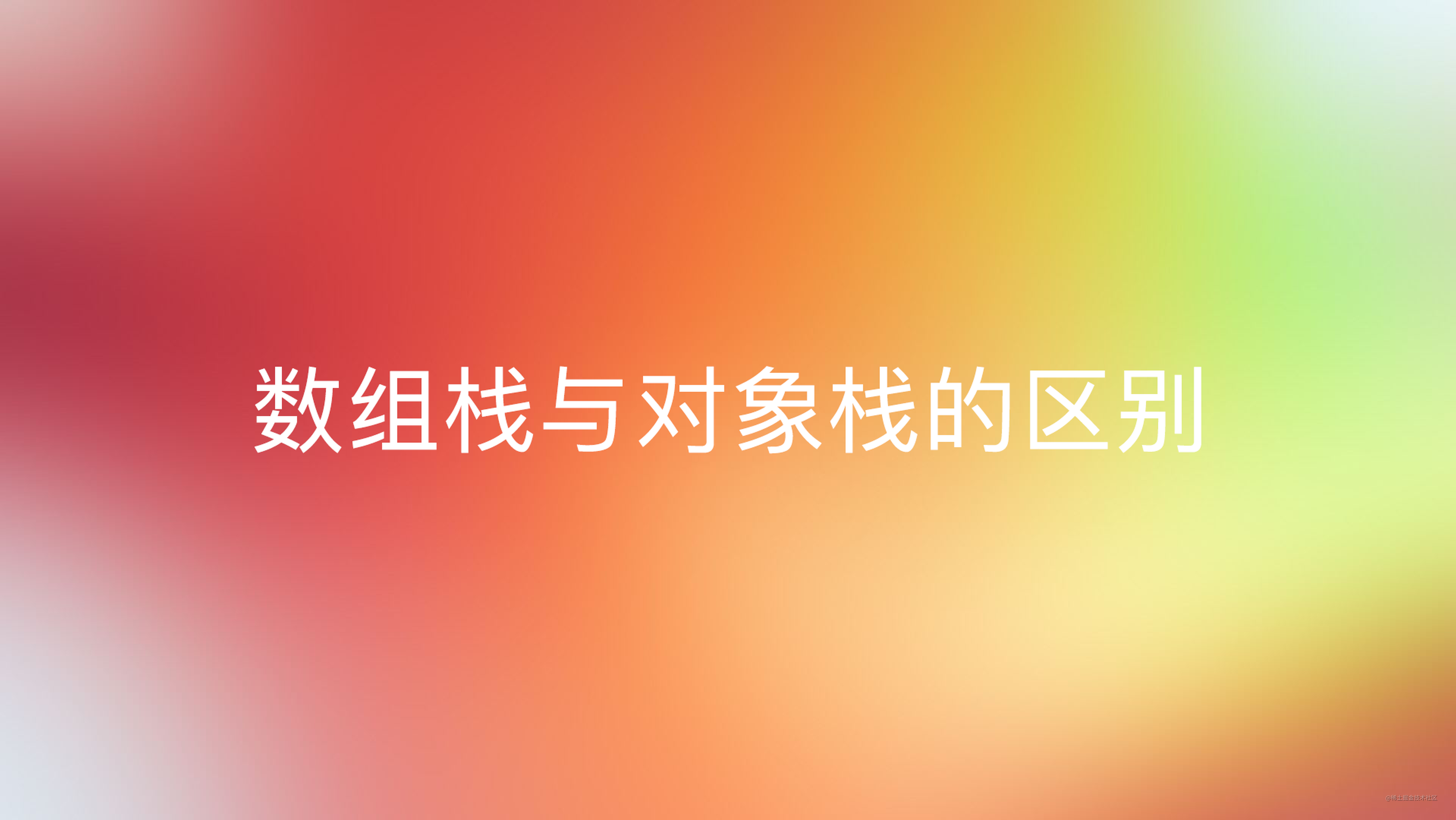# 数组实现栈与对象实现栈的区别## 数组实现栈

### 实现思路

• 入栈，添加一个新元素至栈顶（数组的末尾）。
• 出栈，将栈顶的元素移除并返回被移除的元素。
• 获取栈顶元素，获取当前栈顶元素返回。
• 判断栈是否为空，判断栈（数组）内是否有数据。
• 清空栈，移除栈内所有的元素。
• 获取栈大小，返回栈里的元素个数。
• 输出栈内数据，将栈中的所有元素以字符串的形式返回。

• 入栈(push)，可以使用数组的push方法直接往数组的末尾添加元素。
• 出栈(pop)，可以使用数组的pop方法直接移除栈中的元素，该方法会返回当前被移除的元素。
• 栈顶元素(peek)，可以通过数组的长度-1获取到数组中的最后一个元素。
• 栈是否为空(isEmpty)，可以通过判断数组的长度是否为0来实现。
• 清空栈(clear)，可以将数组直接赋值为空或者调用出栈方法直至栈中的数据为空。
• 栈大小(size)，可以返回数组的长度。
• 输出栈内数据，可以调用数组的toString方法将数组转换为字符串。

### 实现代码

• 新建一个Stack.ts文件
• 定义栈并规定其类型
``````    private items: any[];

• 在构造器中初始化栈
``````    constructor() {
this.items = [];
}

• 根据实现思路实现栈中的函数
``````    // 入栈
push(item:any) {
this.items.push(item);
}
// 出栈
pop() {
return this.items.pop();
}
// 返回栈顶元素
peek() {
return this.items[this.items.length - 1];
}
// 判断栈是否为空
isEmpty() {
return this.items.length === 0;
}
// 清空栈栈内元素
clear() {
this.items = [];
}
// 获取栈内元素数量
size():number{
return this.items.length;
}
// 将栈内元素转为字符串
toString(){
return this.items.toString();
}

### 编写测试代码

• 新建一个StackTest.js文件
• 实例化一个栈
``````const stack = new Stack();

• 测试栈内方法是否正确执行
``````// 入栈
stack.push("第一条数据");
stack.push("第二条数据");

// 出栈
stack.pop();

// 返回栈顶元素
console.log(stack.peek());

// 查看栈大小
console.log(stack.size());

// 判断栈是否为空
console.log(stack.isEmpty());

// 返回栈内所有元素
console.log(stack.toString())

// 清空栈
stack.clear()

## 对象实现栈

### 实现代码

• 新建一个ObjStack.ts文件
• 定义栈对象结构
``````interface StackObj {
[propName: number] : any;
}

• 定义栈并规定其类型，count用于记录栈的大小。
``````private items: StackObj;
private count: number;

• 在构造器中初始化栈相关变量
``````this.items = {};
this.count = 0;

• 入栈，当前栈的大小为新元素的key。
``````push(item: any) {
this.items[this.count] = item;
this.count++;
}

• 出栈，当前栈大小-1，取出栈顶元素，删除栈顶元素，返回取出的栈顶元素
``````pop() {
if(this.isEmpty()){
return undefined;
}
this.count--;
const result = this.items[this.count];
delete this.items[this.count];
console.log(this.items);
return result;
}

• 返回栈顶元素，以当前栈大小-1为key获取其对应的value值。
``````peek() {
if(this.isEmpty()){
return undefined;
}
return this.items[this.count - 1];
}

• 判断栈是否为空，清空栈内元素，获取栈内元素数量
``````// 判断栈是否为空
isEmpty() {
return this.count === 0;
}
// 清空栈内元素
clear() {
this.items = [];
this.count = 0;
}
// 获取栈内元素数量
size():number{
return this.count;
}

• 将栈内元素转为字符串，遍历当前栈对象中的数据，将栈中的数据用逗号拼接并返回。
``````toString(){
if (this.isEmpty()){
return "";
}
let objString = `\${this.items}`;
for (let i = 1; i < this.count; i++){
objString = `\${objString},\${this.items[i]}`
}
return objString;
}

### 编写测试代码

• 新建一个StackObjTest.js文件
• 实例化一个栈
``````const stack = new ObjStack();

• 测试栈内方法是否正确执行
``````// 入栈
stack.push("第一条数据");
stack.push("第二条数据");

// 出栈
stack.pop();

// 返回栈顶元素
console.log(stack.peek());

// 查看栈大小
console.log(stack.size());

// 判断栈是否为空
console.log(stack.isEmpty());

// 返回栈内所有元素
console.log(stack.toString())

// 清空栈
stack.clear()

## 二者的区别

### 十进制转二进制

• 声明一个函数参数为一个十进制数
``````const decimalToBinaryStack = function (decNumber) {

}

• 函数内部声明一个变量，用于接收当前传进来的参数进行除法运算后得到的值。
``````// 传进来的十进制数
let number = decNumber;

• 函数内部实例化一个栈，用于保存模运算后得出的值。
• 函数内部声明两个变量，用户保存当前模运算的值和最终生成的二进制字符串
``````// 余数
let rem;
// 二进制结果
let binaryString = "";

• while循环，判断当前参数进行除法运算后得到的值是否为0，如果不为0就对当前结果进行模运算，将模运算得到的值入栈，对当前结果进行除法运算，直至当前结果为0。
``````while (number > 0) {
// 模运算
rem = Math.floor(number % 2);
// 将余数入栈
stack.push(rem);
// 当前十进制结果除以二
number = Math.floor(number / 2);
}

• while循环，将栈中的数据取出拼接到二进制结果字符串中去
``````while (!stack.isEmpty()) {
binaryString += stack.pop().toString();
}

• 返回二进制结果字符串
``````return binaryString;

## 写在最后

• 文中如有错误，欢迎在评论区指正，如果这篇文章帮到了你，欢迎点赞和关注😊
• 本文首发于掘金，未经许可禁止转载💌